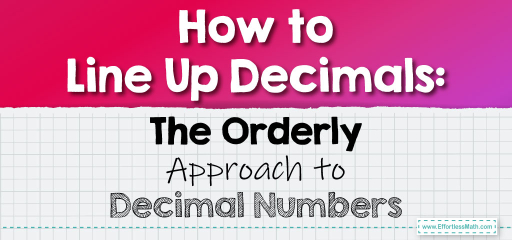# How to Line Up Decimals: The Orderly Approach to Decimal Numbers

Ordering decimals can be a bit like arranging puzzle pieces. It requires careful attention to each digit's place value. Whether you're arranging decimals from least to greatest or vice versa, understanding the value of each position is key. Let's explore the steps to put decimal numbers in order.## Putting Decimal Numbers in Order

### Example 1:

Decimals to Order: $$0.5$$, $$0.58$$, $$0.55$$

Ordering Process:

1. Compare the tenths place: All have $$5$$.

2. Compare the hundredths place: $$0.5$$ has none, $$0.58$$ has $$8$$, and $$0.55$$ has $$5$$.

3. Arrange from least to greatest based on the hundredths place.

Ordered Decimals:

$$0.5$$, $$0.55$$, $$0.58$$

The Absolute Best Book for 5th Grade Students

### Example 2:

Decimals to Order: $$0.72$$, $$0.725$$, $$0.7$$

Ordering Process:

1. Compare the tenths place: All have $$7$$.

2. Compare the hundredths place: $$0.72$$ has $$2$$, $$0.725$$ has $$2$$, and $$0.7$$ has none.

3. For $$0.72$$ and $$0.725$$, compare the thousandths place.

4. Arrange from least to greatest.

Ordered Decimals:

$$0.7$$, $$0.72$$, $$0.725$$

Ordering decimals requires careful attention to each digit’s value. By systematically comparing each place value, you can confidently arrange any set of decimals. This skill is not only academically valuable but also practical in real-world scenarios like shopping, banking, and more. Keep practicing to master the art of ordering decimals!

### Practice Questions:

1. Order the decimals $$0.45$$, $$0.5$$, and $$0.455$$ from least to greatest.

2. Arrange $$0.9$$, $$0.91$$, and $$0.89$$ from greatest to least.

3. Determine the order for $$0.123$$, $$0.13$$, and $$0.12$$ from least to greatest.

4. Which comes first in ascending order: $$0.505$$, $$0.51$$, or $$0.5$$?

5. Order $$0.67$$, $$0.670$$, and $$0.675$$ from greatest to least.

A Perfect Book for Grade 5 Math Word Problems!

1. $$0.45$$, $$0.455$$, $$0.5$$

2. $$0.9$$, $$0.91$$, $$0.89$$

3. $$0.12$$, $$0.123$$, $$0.13$$

4. $$0.5$$

5. $$0.675$$, $$0.67$$ or $$0.670$$ (they are equal), $$0.67$$

The Best Math Books for Elementary Students

### What people say about "How to Line Up Decimals: The Orderly Approach to Decimal Numbers - Effortless Math: We Help Students Learn to LOVE Mathematics"?

No one replied yet.

X
51% OFF

Limited time only!

Save Over 51%

SAVE $15 It was$29.99 now it is \$14.99